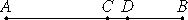# Proposition 25

If an odd number is subtracted from an even number, then the remainder is odd.

Let the odd number BC be subtracted from the even number.

I say that the remainder CA is odd.VII.Def.7

Subtract the unit CD from BC, therefore DB is even.

But AB is also even, therefore the remainder AD is also even. And CD is a unit, therefore CA is odd.

Therefore, if an odd number is subtracted from an even number, then the remainder is odd.

Q.E.D.

## Guide

This is the second of four propositions that examine the result of subtracting even and odd numbers from each other.